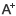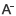# quantityexercise:HypothesisTesting日期：2020-10-23     来源：www.36001.cn    作者：智学网    浏览：456

Questions 1:

If a paired comparison test of mean differences supports rejecting the nullhypothesis, then the:

A 、independence of the samples is statistically significant.

B 、standard error of the mean differences is low relative to the sample meandifference.

C、 difference in means is not statistically significant.

Questions 2:

A tree diagram is most likely used when dealing with investment problems thatinvolve outcomes that are:

A、 independent at each node.

B 、mutually exclusive.

C、 unconditional at each node.

【analysis】

B is correct. According to the test statistic,

the lower the standard error in the denominator, the higher the value of the t-statistic. The t-statistic calculation includes the sample mean difference in the numerator. Therefore, a lower standard error (denominator) relative to the sample mean difference (numerator) results in a higher t-statistic value.

A is incorrect because a paired comparison test is used to test mean differences when we believe that the samples are dependent; it is not used to test whether samples are dependent or independent.

C is incorrect because if the null hypothesis is rejected, the evidence is statistically significant

【analysis】

B is correct. The following figure depicts an example of a tree diagram:

A tree diagram is a diagram with branches emanating from nodes representing either mutually exclusive outcomes or mutually exclusive decisions. Mutually exclusive outcomes are dependent (the occurrence of one outcome does affect the probability of occurrence of the other outcome). In addition, outcomes at each node are conditional (the probability of an outcome is conditioned on another outcome).

A is incorrect. Two outcomes are independent if the occurrence of one outcome does not affect the probability of occurrence of the other outcome. At each node of a tree diagram, the two outcomes that follow are dependent because the probability on the outcome on one branch is related to the probability of the outcome on the other branch.

C is incorrect. Outcomes are unconditional when the probability of an outcome is not conditioned on another outcome. In a tree diagram, outcomes at each node are conditional (the probability of an outcome is conditioned on another outcome).